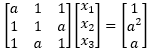# Systems of linear equations

• I
Summary:
What conditions will there be no solutions, a unique solution and a p-parameter of solutions? If possible may you express in particular and homogenous vectors. I'm a bit rusty so bear with me, trying to reestablish my understanding here.StoneTemplePython
Gold Member
I'd strongly suggest multiplying by a permutation matrix ##\mathbf P## so that the LHS matrix is

##\mathbf {11}^T + (a-1)\mathbf I##
and the RHS has the natural progression of ##1, a, a^2 ##

assuming this is in reals, you should be able to confirm that any ##a \neq 1, -2## implies an invertible matrix. And ##a=1## still has (at least one) solution because.... and as for ##a=-2## well...

Hmm why is that you used that expression for the LHS. I am curious, where does the 11^T comes from?

StoneTemplePython
Gold Member
Hmm why is that you used that expression for the LHS. I am curious, where does the 11^T comes from?
because ##\mathbf {11}^T## is the ones matrix and it is easy to work with. The (scaled) Identity matrix is even easier to work with.

and the RHS is most natural as ##1, a, a^2## -- this is called the moment curve.

mathman
No solution when the determinant of the matrix is 0.

StoneTemplePython
Gold Member
No solution when the determinant of the matrix is 0.
This is not correct.

The matrix is rank one when ##a=1## and yet there are ##\gt 0## solutions. e.g. any of the standard basis vectors will do for x here.

I was trying to get OP to come to this conclusion when I said

And ##a=1## still has (at least one) solution because....